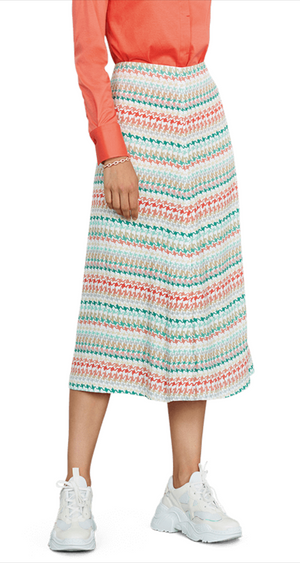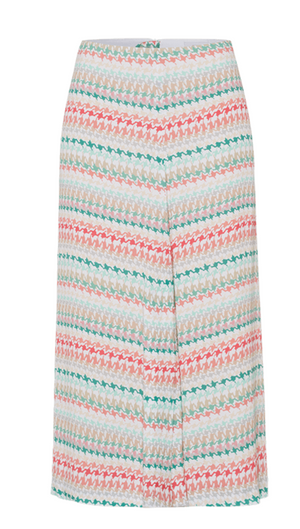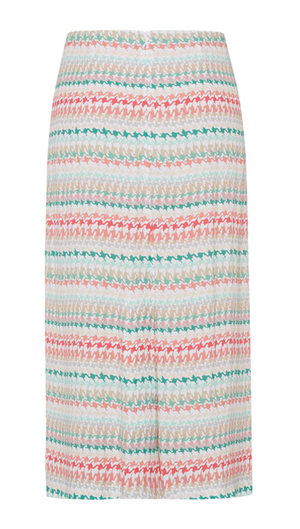Riani

# Printed skirt

Regular price \$495 Unit price  per

Shipping calculated at checkout.
• 100% viscose
• Dry clean only
• Midi length
• Fun spring/summer pattern
• High waist
• A line
• Fits true to size

Size Guide

• 34 = 4 = XS
• 36 = 6 = S
• 38 = 8 = M
• 40 = 10 = M
• 42 = 12 = L
• 44 = 14 = L
• 46 = 16 = XL